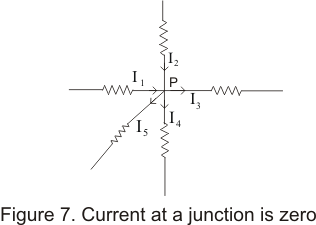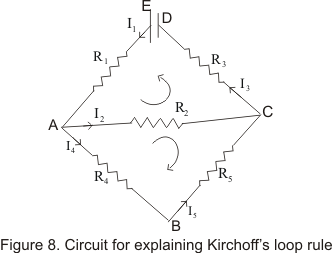# Kirchhoff's Rules

## Kirchhoff's Rules

• We have already analysed simple circuit using ohm's laws and reducing these circuit to series and parallel combination of resistors
• But we also come across circuits containing sources of EMF and grouping of resistors can be far more complex and can not be easily reduced to a single equivalent resistors
• Such complex circuits can be analysed using two Kirchhoff's rules

### (A) The junction Rule (or point rule)

• This law states that "The algebraic sum of all the currents entering junction or any point in a circuit must be equal to the sum of currents leaving the junction"
• Alternatively this rule can also be stated as " Algebraic sum of the currents meeting at a point in a electric circuit is always zero i.e
ΣI=0 at any point in a circuit
• This law is based on the law of conservation of charge
• Consider a point P in an electric circuit at which current I1,I2,I3 and I4 are flowing through conductors in
the direction shown below in the figure below• If we take current flowing towards the junction as positive and current away from the junction as negative,then from Kirchhoff's law
I1+I2+(-I3)+(-I4)=0
or,
I1+ I2=I3+ I4
• From this law ,we conclude that net charge coming towards a point must be equal to the net charge going away from this point in the same interval of time

### (B) The Loop Rule (or Kirchhoff's Voltage Law)

• The rule states that " the sum of potential difference across all the circuit elements along a closed loop in a circuit is zero
ΣV=0 in a closed loop
• Kirchhoff's loop rule is based on the law of conservation of energy because total amount of energy gained and lost by a charge round a trip in a closed loop is zero
• when applying this Kirchhoff's loop rule in any DC circuit,we first choose a closed loop in a circuit that we are analysing
• Next thing we have to decide is that whether we will traverse the loop in a clockwise direction or in anticlockwise direction and the answer is that ,the choice of direction of travel is arbitrary to reach the same point again
• When traversing the loop ,we will be following convention to note down drop or rise in the voltage across the resistors or battery
(i) If the resistor is being traversed in the direction of the current then change in PD across it is negative i.e -IR
(ii)If the resistor is being traversed in the direction opposite to the current then change in PD across it is negative i.e IR
(iii) If a source of EMF is traversed in the direction from -ve terminal to its positive terminal then change in electric potential is positive i.e E
(iv)If a source of EMF is traversed in the direction from +ve terminal to its negative terminal then change in electric potential is negative i.e -E
• We would now demonstrate the use of Kirchhoff's loop law in finding equations in simple circuit
• Consider the circuit as shown below• First consider loop ABDA.Lets traverse loop in anticlockwise direction.From Kirchhoff's loop law
ΣV=0
• Neglecting internal resistance of the cell and using sign conventions stated previously we find
-I3R3+E-I1R1-I2R2=0
or
I1R1+I2R2+I3R3=E
And similarly if we traverse the loop ABCA in clock wise direction
-I2R2+I5R5+I4R4=0
or,
I5R5+I4R4-I2R2=0